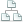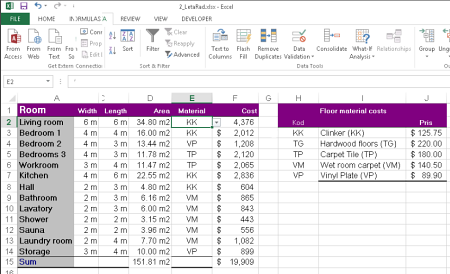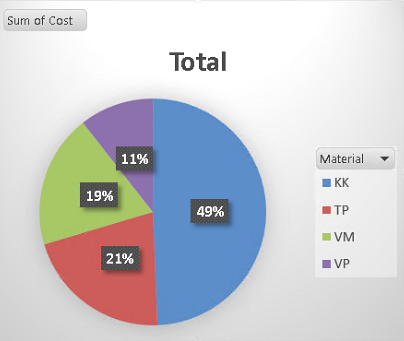Home >Tips >Excel cost calculationsSite map

Calculate, compare and visualize costs in Excel 2013

An Excel tutorial by Peter KalmstromThe possibility to tell Excel to calculate is a crucial feature and the most important reason to use it. Another reason for Excel's popularity is that you can visualize data in charts.

In the demo below Peter Kalmstrom calculates the costs for different kinds of flooring materials and shows the result in a graph.While setting up the comparison between flooring materials Peter gives many useful tips. He shows how to:
• Set number format
• Create a multiply formula
• Name an array of cells
• Create a vertical lookup formula
• Use the AutoSum button
• Copy format
• Create a data validation rule
• Create a pivot chartPeter uses Excel 2013 for his demo, but most of what he shows can be done in earlier versions of Excel too. If you use SharePoint 2010, Peter's demo on holiday calculations might be of interest.